603 - 5885 1250 (4 lines) info@mes100.com
Select Page

Reference:

• MSMA 2nd Edition; Chapter 2; 2.5 Hydrological Routing
• MSMA 2nd Edition; Chapter 7 – Detention Pond

#### How to perform routing through a reservoir or storage facility using Equation 2.17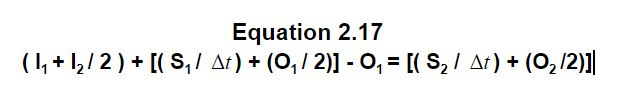#### Step 1:

Develop:

i. Inflow hydrograph

• Rational Method refer Chapter 2; 2.3.2 Rational Hydrograph Method)
• Time Area Method refer Chapter 2;  2.3.3 Time Area Hydrograph Method)
• Refer Chapter 7; 7.5.2 Stage Storage Curves Development
• Refer Chapter 7; 7.5.3 Stage Discharge Curves

For the proposed facility (pond)

#### Step 2:

Select a routing time period (t); and provide a minimum of five (5) points on the rising limb of the inflow hydrograph

#### Step 3:

Develop ‘storage indicator numbers’ table from ‘stage-discharge curve’ & ‘stage-storage curve’

Typical storage indicator numbers table contains the following column headings;Notes;

• Discharge (O) and stage (S) – obtained from stage-discharge & stage-storage curves
• Subscript 2 is arbitrarily assigned in the table
• Time interval t must be the same interval used in the tabulated inflow hydrograph

#### Step 4:

Storage indicator number curves are plotted by:

the outflow (column 2) vertically against the storage indicator numbers in column 6.#### Step 5:

Supplementary curve of storage: Column 3 vs Column 6 (S2 /t + O2 /2)

*refer figure 2.12 above.

Purpose: useful for identifying storage for any given value of S2 /t + O2 /2.

#### Step 6:

Now routing can be performed by developing a routing table for the solution of Equation 2.17 as follows:##### Column 1 & 2Note:

To calculate Inflow (Column 2); user can used equation 2.3; Q = CiA / 360

##### Column 3##### Column 4,5,6 & 7 (at time 0min )##### Column 6

Referring to Equation 2.17;

• From equation 2.17; the left side of equation is determined algebraically as columns (3) + (4) – (5). And this value equals the right side of the equation and is placed in column (6)Note:

• Discharge (O) and storage (S) are obtained from the stage-discharge and stage-storage curves respectively
• Time interval (t) must be the same as the time interval used in the tabulated inflow hydrograph
##### Column 7

Enter the storage indicator curve with S2 / t + O2 / 2 (column 6) to obtain O2 (column 7)

Used Stage-Discharge Relation and Storage Indicator Numbers table to calculate O2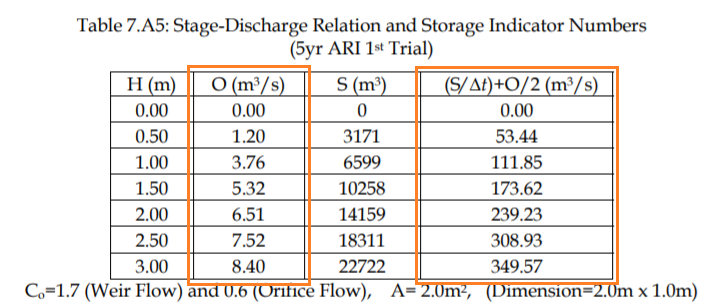For example

take t = 9min ;  S2 / t + O2 / 2 = 5.88 m3/s

So interpolate;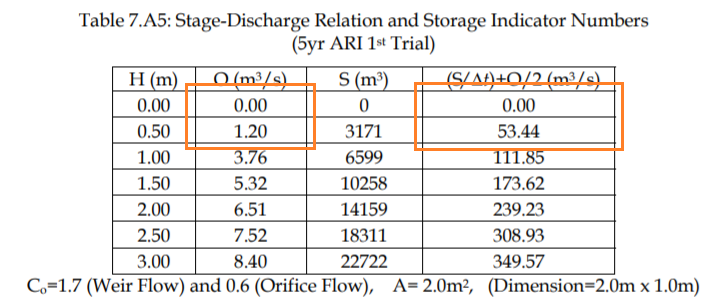Hence the O2 at t= 9min is 0.12 m3/s

(refer image below for reference)#### Next is how to proceed the pond routing?

Column 6 (S2 / t + O2 / 2) and Column 7 (O2 ) are transported to the next line and become the Column 4 (S1 / t + O1 / 2) and Column 5 (O1 ) respectively;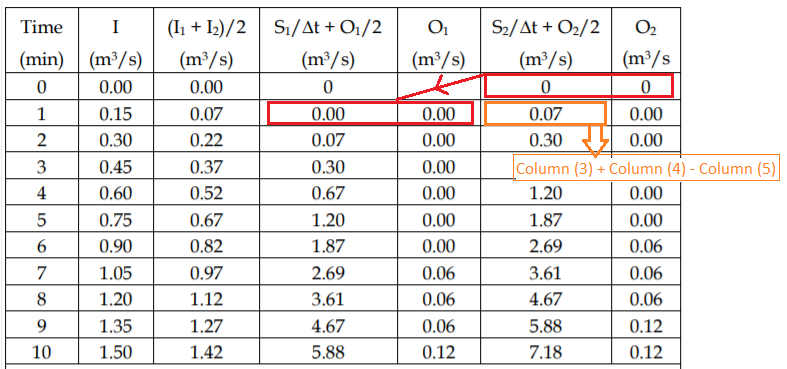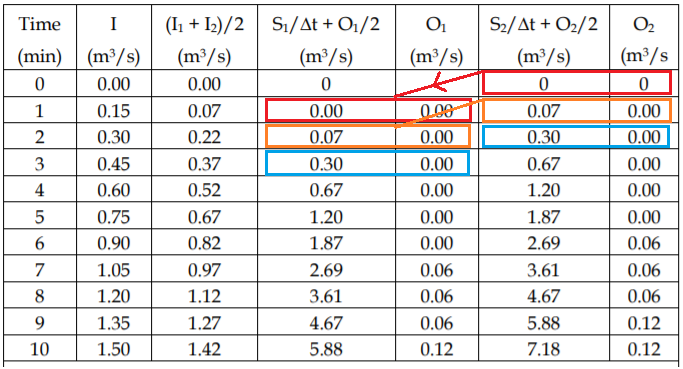Notes:

1. Peak storage depth and discharge (O2  in Column (7) will occur when Column (6) reaches maximum.
2. The storage indicator numbers table developed in is entered with the maximum value of S2 / t + O2 / 2 to obtain the maximum amount of storage required. The table also can be used to determine the corresponding elevation of the depth of stored water.
3. Designer needs to make sure that the peak flow in Column (7) does not exceed the allowable discharge as prescribed by the stormwater management criteria.

#### Step 7:

Plot outflow hydrograph; O2 Column (7) vs time Column (1)